# Minors of a $3 \times 3$ matrix

The determinant of the square sub-matrix of the order two by leaving the row and the column of an element is called the minor of that entry in the square matrix of the order three.

## Introduction

A three by three square matrix contains nine entries, which are arranged in three rows and three columns. For calculating the minor of an element, the remaining entries in that row and column are neglected. It forms a two by two square matrix with the remaining entries and the determinant of this matrix is called the minor of the respective entry. Thus, nine minors can be calculated for the nine elements in a matrix of the order $3$.

$D$ $\,=\,$ $\begin{bmatrix} d_{11} & d_{12} & d_{13} \\ d_{21} & d_{22} & d_{23} \\ d_{31} & d_{32} & d_{33} \\ \end{bmatrix}$

Now, let’s learn how to find the minor of every element in the matrix $D$ of the order $3 \times 3$. In order to find the minors of entries in a matrix of the order $3$, the knowledge on finding the determinant of a second order matrix is required.

### Minors for the first row entries

Look at the entries in the first row of the matrix $D$. The entries $d_{11}$, $d_{12}$ and $d_{13}$ are the elements in the first row of the matrix $D$. The minors of them are denoted by $M_{11}$, $M_{12}$ and $M_{13}$ respectively. Now, let’s learn how to find the minor of each element in the first of the $3 \times 3$ matrix.

#### Minor of the entry in first row and first column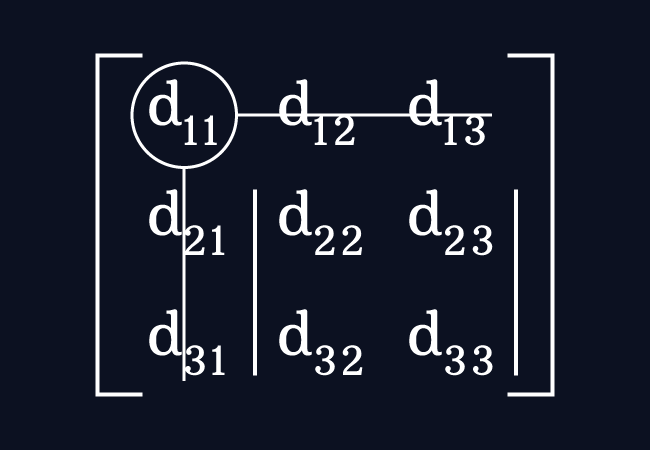The entry $d_{11}$ is an element in the first row and first column. Leave the elements in the first row and the entries in the first column. It forms a square matrix of the order $2$ by the remaining elements.

Then, find the determinant of the matrix for calculating the minor of the entry $d_{11}$ and it is denoted by $M_{11}$ in matrix algebra.

$M_{11}$ $\,=\,$ $\begin{vmatrix} d_{22} & d_{23} \\ d_{32} & d_{33} \\ \end{vmatrix}$

$\therefore\,\,\,$ $M_{11}$ $\,=\,$ $d_{22} \times d_{33}\,-\,d_{23} \times d_{32}$

#### Minor of the entry in first row and second column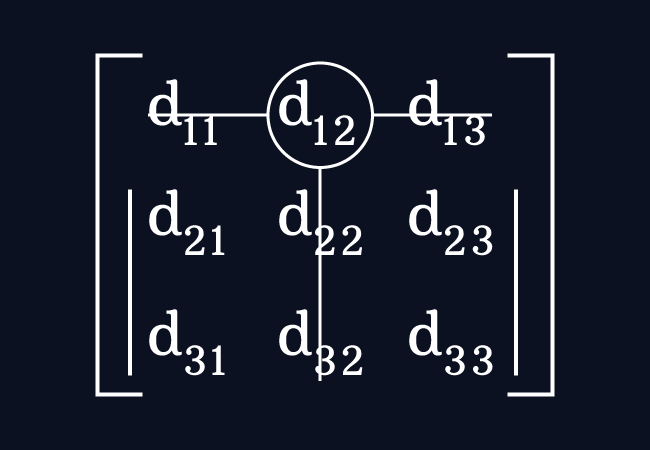The element $d_{12}$ is an entry in the first row and second column. Leave the entries in the first row and the entries in the second column. It forms a square matrix of the order $2 \times 2$ by the remaining elements.

Now, calculate the determinant of the matrix for finding the minor of the element $d_{12}$ and it is denoted by $M_{12}$ in mathematics.

$M_{12}$ $\,=\,$ $\begin{vmatrix} d_{21} & d_{23} \\ d_{31} & d_{33} \\ \end{vmatrix}$

$\therefore\,\,\,$ $M_{12}$ $\,=\,$ $d_{21} \times d_{33}\,-\,d_{23} \times d_{31}$

#### Minor of the entry in first row and third column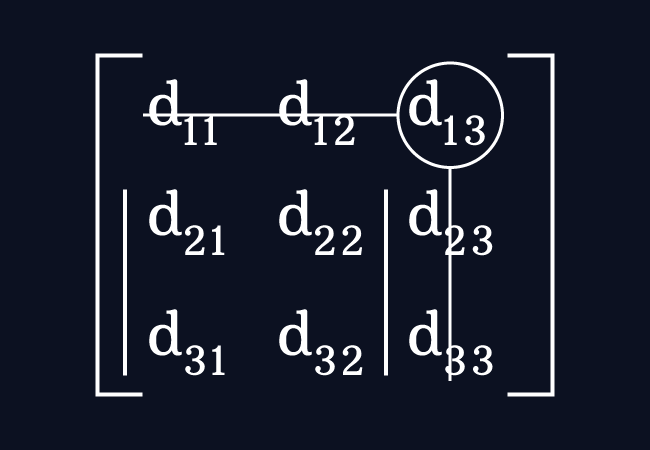The entry $d_{13}$ is an element in the first row and third column. Leave the elements in the first row and the entries in the third column. It forms a second order square matrix by the remaining elements.

Now, find the determinant of the matrix for evaluating the minor of the entry $d_{13}$ and it is simply represented by $M_{13}$ in matrices.

$M_{13}$ $\,=\,$ $\begin{vmatrix} d_{21} & d_{22} \\ d_{31} & d_{32} \\ \end{vmatrix}$

$\therefore\,\,\,$ $M_{13}$ $\,=\,$ $d_{21} \times d_{32}\,-\,d_{22} \times d_{31}$

### Minors for the second row elements

Focus on the entries in the second row of the matrix $D$. The elements $d_{21}$, $d_{22}$ and $d_{23}$ are the entries in the second row of the matrix $D$. The minors of those elements are represented by $M_{21}$, $M_{22}$ and $M_{23}$ respectively. Now, let’s learn how to find the minor of every entry in the second row of the third order matrix.

#### Minor of the entry in second row and first column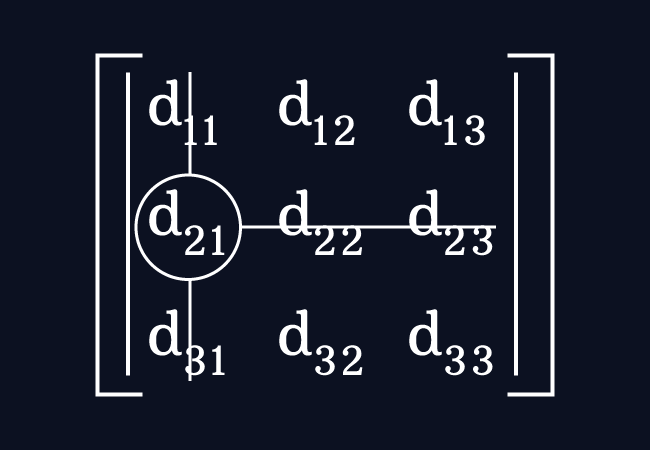The element $d_{21}$ is an entry in the second row and first column. Leave the elements in the second row and the elements in the first column. It forms a matrix of the order $2 \times 2$ by the remaining elements.

Now, find the determinant of the matrix for evaluating the minor of the entry $d_{21}$ and it is denoted by $M_{21}$ in this matrix algebra.

$M_{21}$ $\,=\,$ $\begin{vmatrix} d_{12} & d_{13} \\ d_{32} & d_{33} \\ \end{vmatrix}$

$\therefore\,\,\,$ $M_{21}$ $\,=\,$ $d_{12} \times d_{33}\,-\,d_{13} \times d_{32}$

#### Minor of the entry in second row and second column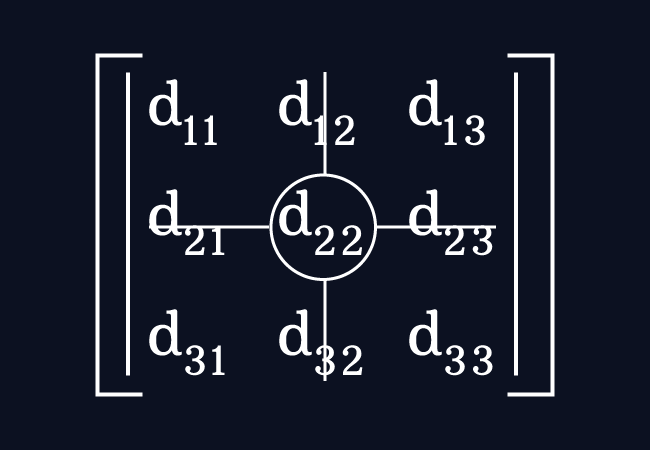The element $d_{22}$ is an element in the second row and second column. Neglect the entries in the second row and the entries in the second column. It forms a second order square matrix by the remaining elements.

Then, evaluate the determinant of the matrix to find the minor of the element $d_{22}$. In mathematics, it is represented by $M_{22}$.

$M_{22}$ $\,=\,$ $\begin{vmatrix} d_{11} & d_{13} \\ d_{31} & d_{33} \\ \end{vmatrix}$

$\therefore\,\,\,$ $M_{22}$ $\,=\,$ $d_{11} \times d_{33}\,-\,d_{13} \times d_{31}$

#### Minor of the entry in second row and third column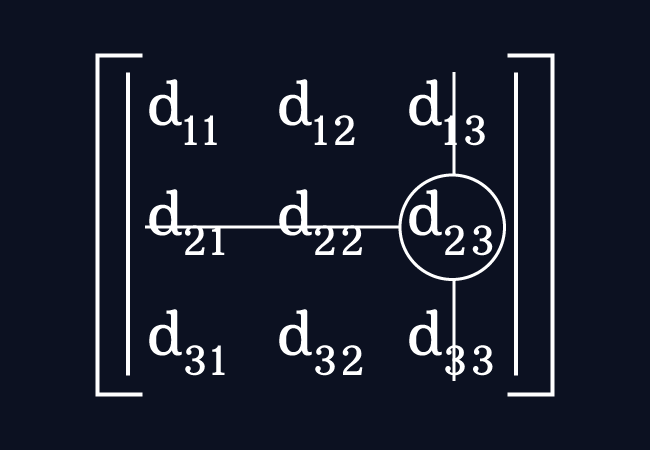The entry $d_{23}$ is an element in the second row and third column. Now, leave the elements in the second row and the entries in the third column. Thus, it forms a square matrix of the order $2 \times 2$ by the remaining elements.

Find the determinant of the matrix to find the minor of the element $d_{23}$ and it is denoted by $M_{23}$ simply in matrices.

$M_{23}$ $\,=\,$ $\begin{vmatrix} d_{11} & d_{12} \\ d_{31} & d_{32} \\ \end{vmatrix}$

$\therefore\,\,\,$ $M_{23}$ $\,=\,$ $d_{11} \times d_{32}\,-\,d_{12} \times d_{31}$

### Minors for the third row entries

Finally, concentrate on the elements in the third row of the matrix $D$. The elements $d_{31}$, $d_{32}$ and $d_{33}$ are the elements in the third row of the matrix $D$. The minors of those entries are denoted by $M_{31}$, $M_{32}$ and $M_{33}$ respectively. Now, let’s know how to evaluate the minors of them in the third row of the matrix.

#### Minor of the entry in third row and first column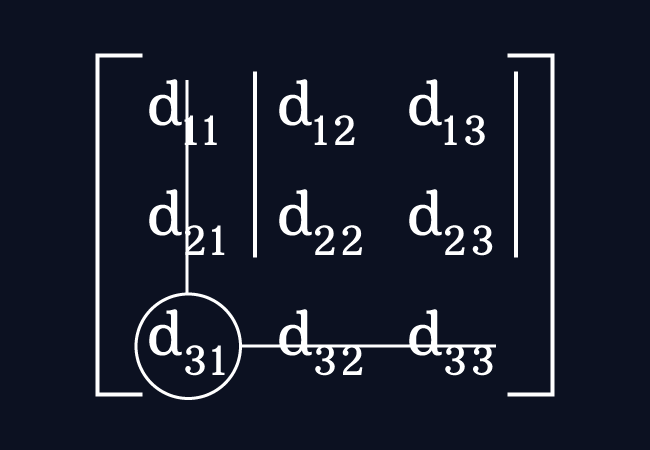The element $d_{31}$ is an element in the third row and first column. Leave the entries in the third row and the entries in the first column. It forms a $2 \times 2$ square matrix by the remaining elements.

Then, find the determinant of the matrix for evaluating the minor of the entry $d_{31}$ and it is denoted by $M_{31}$ in matrix algebra.

$M_{31}$ $\,=\,$ $\begin{vmatrix} d_{12} & d_{13} \\ d_{22} & d_{23} \\ \end{vmatrix}$

$\therefore\,\,\,$ $M_{31}$ $\,=\,$ $d_{12} \times d_{23}\,-\,d_{13} \times d_{22}$

#### Minor of the entry in third row and second column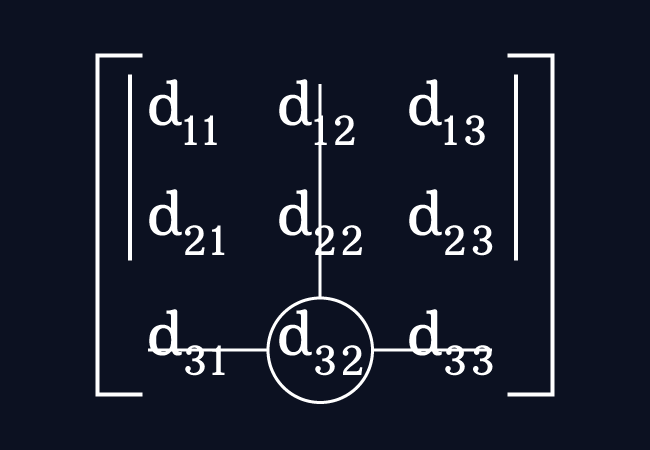The element $d_{32}$ is an entry in the third row and second column. Neglect, the entries in the third row and the entries in the second column. It forms a square matrix of the order $2 \times 2$ by the rest of elements.

Now, evaluate the determinant of the matrix for evaluating the minor of the element $d_{32}$ and it is denoted by $M_{32}$ in mathematics.

$M_{32}$ $\,=\,$ $\begin{vmatrix} d_{11} & d_{13} \\ d_{21} & d_{23} \\ \end{vmatrix}$

$\therefore\,\,\,$ $M_{32}$ $\,=\,$ $d_{11} \times d_{23}\,-\,d_{13} \times d_{21}$

#### Minor of the entry in third row and third column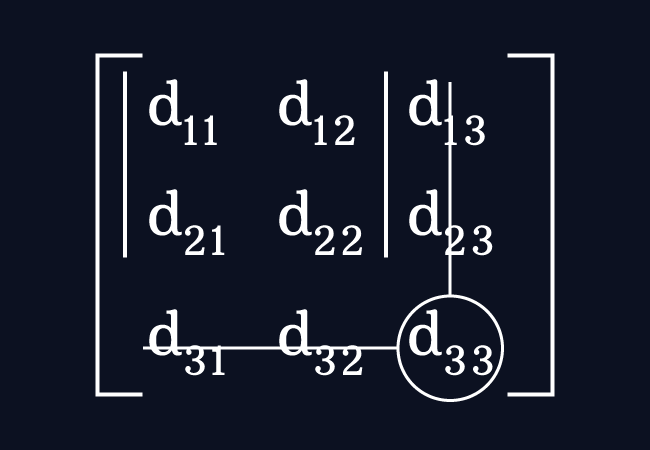The entry $d_{33}$ is an element in the third row and third column. Leave the elements in the third row and the entries in the third column. It forms a second order square matrix by the rest of the elements.

Now, calculate the determinant of the matrix in order to evaluate the minor of the entry $d_{33}$ and it is represented by $M_{33}$ simply in matrices.

$M_{33}$ $\,=\,$ $\begin{vmatrix} d_{11} & d_{12} \\ d_{21} & d_{22} \\ \end{vmatrix}$

$\therefore\,\,\,$ $M_{33}$ $\,=\,$ $d_{11} \times d_{22}\,-\,d_{12} \times d_{21}$

Latest Math Topics
Jun 26, 2023
Jun 23, 2023

Latest Math Problems
Jul 01, 2023
Jun 25, 2023
###### Math Questions

The math problems with solutions to learn how to solve a problem.

Learn solutions

Practice now

###### Math Videos

The math videos tutorials with visual graphics to learn every concept.

Watch now

###### Subscribe us

Get the latest math updates from the Math Doubts by subscribing us.